Document Type : Original Article

Author

a Department of Chemistry, Mahshahr Branch, Islamic Azad University, Mahshahr, Iran

Abstract

The effect of the adsorbed thiophene (T) on the surface of (8,0) zigzag single walled boron nitride nanotubes (BNNTs) was studied using density functional theory calculations in the gas phase. Geometry optimizations were also carried out at the B3LYP/6-31G (d) level of theory. The Gaussian 09 suites of programs were used. The geometric optimization of (8, 0) BNNT-T was performed using the minimum energy criterion in six different configurations of the adsorbed thiophene on the nanotube. Our computer simulations have found that the preferred adsorption site of the molecule is at the end of the nanotube for the T component and all cases have physical interactions. The results showed an increase in polarity due to the proper distribution of electrons. It was also found that the reduction in global hardness, energy gap and electronic chemical potential due to thiophene adsorption leads to an increase in the stability of the (8,0) zigzag BNNT-T complex. In this study, natural bond analysis, global softness, ionization potential and electrophilicity index for nanotubes were calculated.

Graphical Abstract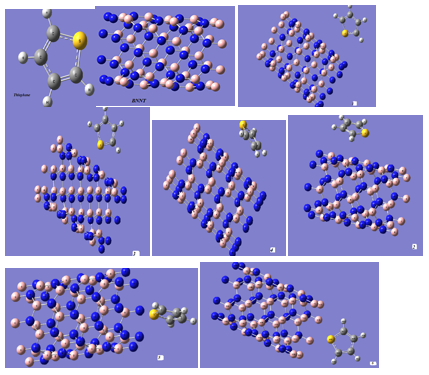Keywords

###### ##### Full Text

Introduction

Boron nitride nanotubes (BNNT) were experimentally synthesized in 1995 [1-2]. Boron is the only known element to form cage molecule clusters and tetragonal structure. Due to the polar nature of B-N bonds, BNNTs nanostructures are expected to have a higher reactivity than their carbon structure [3,4] The BNNTs have special features such as short and open length that make these types of systems suitable for biological applications [3,4]. The BNNTs are semiconductors with wide band gaps and independent of tube diameter and helicity [5,6] that have higher chemical and thermal stability than carbon nanotubes (CNT) . These nanostructures have been used for storage as well as detection of hydrogen molecules [8,9]. Theoretical studies [10-15] have shown variation in the magnetic and electronic properties of both nano tubes and nano sheets made up of BN when these structures interact with organic molecules or functional groups. Thiophene is a -conjugated heterocyclic compound with the molecular formula C4H4S consisting of a planar five-membered ring [3,4]. On the other hand, thiophene molecule (T; C4H4S), which belongs to heterocyclic molecules, is present in petroleum products [16,17]. Based on the presenceof delocalized electrons in sulfur and adjacent carbons 1 and 2, we can point to the high reactivity of this molecule (Figure 1). It is possible to change the electronic properties of nanotubes for technological applications. Mills et al. studied the adsorption reactions of thiophene upon Mo2N/ Al2O3 catalysts using FT-IR spectroscopy. The experimental studies also showed the reactive adsorption of thiophene on Ni/ZnO structure. Denis and Iribarne studied thiophene adsorption on single wall carbon nanotubes and graphene using the VDW-DF and LDA functionals . Peyghan et al. studied adsorption of thiophene on the pristine(6,0) AlN nanotubes in the gas phase using DFT calculations  and also Peyghane et al. studied electronic structure and adsorption process of imidazole on (6,0) zigzag boron nitride nanotube . Baei et al. investigated the adsorption of thiophene over Zn12O12 nanocage structure . Chigo Anota et al. studied heterocyclic molecules interactions .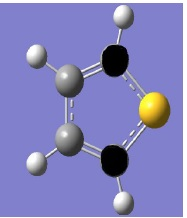Figure 1. Exhibition delocalized electrons on sulfur atom and adjacent carbons 1 and 2

We studied thiophene adsorption on the SWBNNT surface with 6 different configurations. Moreover, we researched the quantum molecular descriptors [18,19], energy gap, global softness (s), electronic chemical potential ( ), global hardness ( ), electrophilicity index ( ),  and electronegativity ( ) of the nanotubes.

Simulation models and computational details

The DFT method is the standard model in many Gamess and Gaussian computational chemistry software systems. For modeling, this research used an 8-core computer system with 500GB of memory and a 2.3 GHz processor running Windows 7. Calculations were performed by Gaussian09. Calculations were based on the density functional theory (DFT) because this method has a higher calculation speed compared to other methods with the same accuracy. The B3LYP method and the basic set of 6-31G (# B3LYP/6-31G) were used for optimization of structures and calculation of interaction energy. The Gaussian software was used to prepare input data and perform calculations. Using a series of graphic softwares such as Nanotube Modeler, HyperChem and GaussView, the desired molecular geometry structure was first drawn. Then, necessary commands were applied to determine the required calculations. Finally, Gaussian09 was introduced as the input file, and in the next Gaussian step, calculations were presented as numbers by solving the Schrödinger electron eqaution. BELYP is a hybrid method in which the electron correlation energy is calculated from Equation (1)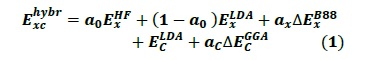In this work, the main calculations of total energy are first performed to investigate the interactions of (8, 0) BNNT with thiophene. To determine the interaction distance between thiophene and nanotubes (Figure 2), we examined six different geometric configurations.

In the first configuration, the thiophene fragment is directed to the boron atom. In the second configuration, the thiophene is placed parallel to the boron atom. In the third, the thiophene is perpendicular to the central hexagon of the nanotube surface; in the fourth, the thiophene is placed parallel to the central hexagon of the nanotube surface; in the fifth, the fragment is perpendicularly oriented to N of the nanotube end; and in the sixth configuration, the thiophene is perpendicularly oriented to B of the nanotubes end.

Neutrality and multiplicity 1, were considered for the aromatic molecule, BNNT and BNNT-thiophene systems. The properties of (8, 0) chiral nanotubes with specifications of length 25nm and diameter 1 A was also studied. The BNNT contains a total of 80 atoms (40N, 40B). The interacting molecule is thiophene. The energy gap is determined as the difference between the HOMO (Highest occupied molecular orbital) and LUMO (lowest   unoccupied molecular orbital) orbital energies. The molecular energy adsorbed on the BN nanotubes is defined as follows: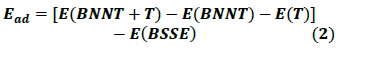The use of the localized basis sets reliably reduces the amount of required computational work when using them with large vacuum regions in the unit cell. However the finiteness of the localized basis sets leads to a basis set superposition error (BSSE) as described by Tournus and Charlier in a study of benzene on CNT . E (BSSE) is defined as the basis set superposition errors. For measuring the chemical reactivity, we determine the chemical potential as the arithmetic average  which shows that the chemical potential of free electron gas is equal to the fermi level and it is considered as the center of the energy gap .

The work function is defined as the minimum required energy for removal of an electron from a solid to a point immediately outside the solid surface or the energy required for transfer of an electron from the fermi energy level into the vacuum. This work function is determined as the energy difference between the vacuum level (LUMO orbital) and the fermi level (chemical potential). Work function was calculated and it is equal to 0.393eV.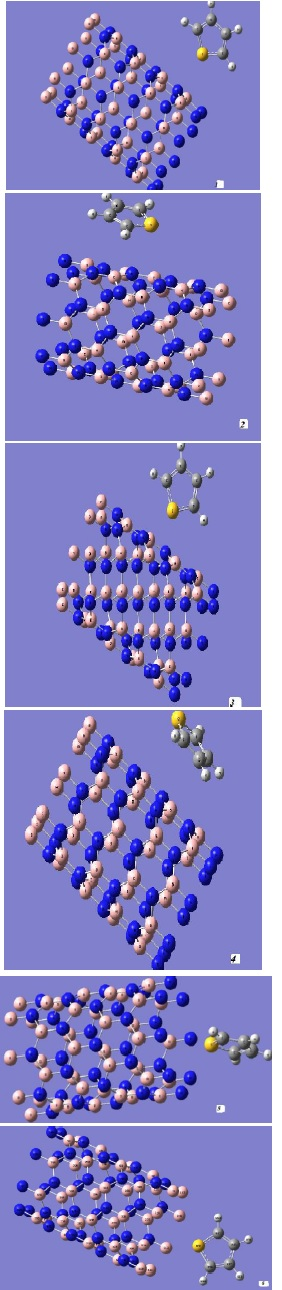Figure 2. Six different geometrical configuration BNNT-T complexes

Results and Discussion

The geometric optimization (Table 1-a,b,c) was performed using previously defined criteria (Figure 3). The results indicated that the atomic structure of the interaction between the BNNT and  fragment is in the most stable state in configuration 6, as shown in Table 2. The aim of the present paper is to gain the quantum molecular descriptors and adsorption properties of the nanotube system. The systems studied are BNNT-thiophene, BNNT, thiophene, ) Figure 3( that exhibit a semiconductor behavior with energy gap values (HOMO-LUMO energy difference) (  eV), ( eV),  eV). The system with BNNT-T exhibited a gap reduction. The polarity increased from for BNNT to  for BNNT-T. The electrical conductivity changes are calculated with formula (3), where  is the energy gap of the HOMO-LUMO difference,  is the Boltzmann's constant,  is the given temperature  and  is the electrical conductivity of the complex.

According to the above equation, a larger energy gap at a certain temperature leads to a smaller electrical conductivity.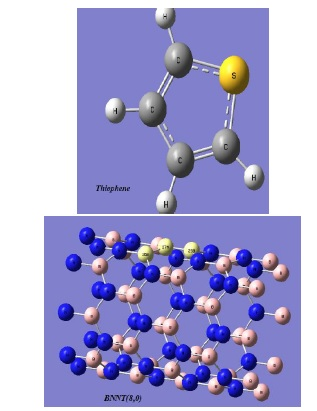Figure 3. The geometric optimization of thiophene molecule and BNNT

Table 1a. The optimal energy of thiophene molecule and BNNT

 Fragment Thiophene BNNT (8,0) -3190.848

Table 1b. The optimum geometrical parameters for the thiophene

 Bond lenght (0A) Thiophene C-H 1/087 C-C 1/42 C-C 1/37 C-S 1/71

Table 1c. The optimum geometrical parameters for the BNNT

 Bond lenght (0A) BNNT N-B(16) 1/45 N-B(25) 1/45

Table 2. Energy of six configuration for BNNT-T complexes

 Configuration   BNT-T 1 -3739.0224 2 -3739.0245 3 -3738.9984 4 -3739.0273 5 -3739.0228 6 -3739.0281

We studied adsorption behavior of thiophene on the SWBNNT with the (8, 0) zigzag SWBNNT model consisting of (B40N40) atoms. In the first step, the structures were allowed to relax using all atomic geometrical parameters in the optimization at the DFT level of B3LYP exchange- functional and 6-31G (d) standard basis set. We calculated the adsorption energy (  as follows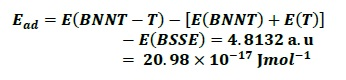For the nanotubes, quantum molecular descriptors [16-17], electronic chemical potential , global hardness , electrophilicity index  , energy gap and global softness (s) were calculated as follows: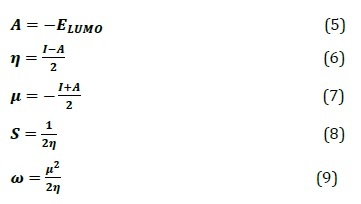where ) is the ionization potential and ) is the electron affinity of the molecule. The electrophilicity index is a measure of the electrophilic power of a molecule. The quantum molecular descriptors were compared for the BNT and thiophene adsorbed on the (8,0) zigzag BNNT (Table 3). All calculations were carried out using the Gaussian 09 suites of programs.

Table 3. The calculated properties for BNNT and BNNT- T complex

 Property BNNT-Ta BNNTb Energy gap 0.00979 0.01034 I 0.20395 0.18305 A 0.194 0.1727 Η 0.00489 0.00517 𝞵 -0.199 -0.1778 S 102.145 96.711 4.045 3.0599

a,b Units are eV

Natural bond orbital analysis

Based on the NBO analysis (Tables 4-7), NHO direction and bending of the bond (deviation from line of nuclear centers) can be estimated to understand the position of  and  orbitals. These data are often useful for predicting the direction of geometry changing results from geometric optimization. The direction of a hybrid is specified in terms of the polar  and azimuthal  angles of the vector describing its p-component. The hybrids directions are then compared with the direction of the center lines between two nuclei to determine the bending of those bonds, which are expressed as the deviation angles between these two directions. For adsorbed thiophene (T) on (8, 0) BNNT-T,  NBO calculations showed that these structures are the dominant Lewis structure. However, based on the advanced valance bond theory, covalent-ionic resonances are not necessary due to the inclusion of bond-polarity effects in a resonance structure. By this work, for each NAO functions, the core, valence or Rydberg, the orbital occupancy and the orbital energy were shown. It was notable that the occupancies of the Rydberg (Ryd) NAOs are typically much lower than those of the core and valence (Val) NAOs of the natural minimum basis (NMB) set.

Electron delocalization can be defined based on Lewis amount. It can be considered that the maximum value of electric charges in the Lewis orbitals represents a low amount of electric charge which corresponds to the strong effects of electron delocalization. In resonance phenomenon, major and minor percentages combinations may appear in several calculations. Based on perturbation theories of Fock Matrix, it is possible to estimate the donor-acceptor (bonding and anti-bonding) interactions in the NBO foundation: These analyses are carried out through examining all possible interactions among types of lewis donors (Field) with (empty) non-Lewis acceptors of NBOs.  Their energy is calculated based on second-order perturbation theories. Since these kind interactions are related to donation of occupancies from the localized Lewis structure to the idealized into the empty non-Lewis orbitals, they are introduced as "delocalization" corrections to the zero-order natural Lewis structures. For any donor (i) and acceptor (j), the stabilization energies E(2) are associated to delocalization. The following equation exhibits the relationship of F Matrix to energy: =  where  is the donor occupancies and    is diagonal orbital energies. In addition,   is the Fock-matrix function.

In quantum chemistry, a natural bond orbital or NBO is a calculated bonding orbital with maximum electron density. The NBOs are one of the sequence of natural localized orbital sets that include "natural atomic orbital" (NAO), "natural hybrid orbital" (NHO), "natural bonding orbital" (NBO) and "natural (semi-)localized molecular orbital" (NLMO). These natural localized sets are intermediate between basis atomic orbital (AO) and molecular orbital (MO):

Atomic orbital → NAO → NHO → NBO → NLMO → Molecular orbital

Natural (localized) orbitals are used in computational chemistry to calculate the electron density distribution in atoms and the bonds between atoms. They have the "maximum-occupancy character" in localized 1-center and 2-center regions of the molecule. Natural bond orbitals (NBOs) include the highest possible percentage of the electron density, ideally providing occupancy close to 2.000, the most accurate possible “natural Lewis structure” of . A high percentage of electron density (shown as %-ρL), which is usually  for typical organic molecules, corresponds to an precise natural Lewis structure.

The concept of natural orbitals was first proposed by Per- Olov Lowdin in 1955, to describe the unique set of orthonormal1-electron functions that are inherently the function of N-electron wave. The bonding NBOs are of the "Lewis orbital"-type (occupation numbers near 2); anti bonding NBOs are of the "non-Lewis orbital"-type (occupation numbers near 0). In an ideal Lewis structure, full Lewis orbitals (two electrons) are complemented by formally empty non-Lewis orbitals. Weak occupancies of the valence anti bonds play the primary role in departures from an ideally localized Lewis structure, which means true "delocalization effects. Lewis optimal structures can be found with a computer program that can calculate NBO. A Lewis optimal structure can be defined as a structure with the maximum amount of electric charge in Lewis orbitals (Lewis load).

A low amount of electric charge in Lewis orbitals indicates strong effects of electron delocalization . Table 4 summarizes a variety of information for each cycle: the occupancy threshold for a "good" pair in the NBO search, the total populations of Lewis and non- Lewis NBOs, the number of core (CR), 2-center bond (BD), 3-center bond (3C), and lone pair (LP) NBOs in the natural Lewis structure, the number of low-occupancy Lewis (L) and high-occupancy (> 0.1e) non-Lewis (NL) orbitals, and the maximum deviation (Dev) of any formal bond order for the structure from a nominal estimate (NAO Wiberg bond index). If all orbitals of the formal Lewis structure cross the occupation threshold, the Lewis structure is accepted ((default = 1.90 electrons).

Table 5 allows one to quickly identify the principal delocalizing acceptor orbitals associated to each donor NBO, and their topological relationship to this NBO, i.e., whether attached to the same atom (geminal, " g"), to an adjacent bonded atom (vicinal, "v"), or to a more remote ("r") site. These acceptor NBOs will generally correspond to the principal "delocalization tails" of the NLMO associated to the parent donor NBO.

NBO calculations using occupation numbers of bonding and non-bonding orbitals, occupation number of Energy, Lewis and non-Lewis, atomic partial charge are given in Tables 4, 5 and 6.

Table 4. Natural bond orbital analysis

 OCCUa LEb STRUCc LOW OCC HIGH OCC Cycle Lewis Non-lewis CR BD 3C LP L NL Dev 1(1) 494.91 29.088 89 121 0 52 51 58 0.64 2(2) 494.91 29.088 89 121 0 52 51 58 0.64 3(1) 495.22 28.77 89 122 0 51 50 58 0.64 4(2) 495.22 28.77 89 122 0 51 50 58 0.64 5(1) 503.109 20.89 89 154 0 19 13 60 0.84 6(2) 503.148 20.85 89 154 0 19 13 59 0.84 7(3) 503.187 20.81 89 154 0 19 13 58 0.35 8(4) 503.187 20.81 89 154 0 19 13 58 0.35

aoccupancies; bLewis; cstructure

Table 5. Occupied number and energy by using NBO calculations for number nitrogen atoms in adsorbed thiophene molecule

 NBO Atom Occupancy E/Kcalmol-1 BD(1) (10)N-N(20) 1.74 -0.24327 (10)N-N(80) 1.73 -0.24341 (20)N-N(30) 1.73 -0.24365 (30)N-N(40) 1.73 -0.24366 (40)N-N(50) 1.74 -0.24353 (50)N-N(60) 1.74 -0.24328 (60)N-N(70) 1.74 -0.24331 CR(1) N(10) 1.99 -14.174 N(20) 1.99 -14.175 N(30) 1.99 -14.173 N(40) 1.99 -14.175 N(50) 1.99 -14.176 LP(1) N(10) 1.95 -0.50647 N(20) 1.95 -0.50600

Table 6. Calculated partial charges by NBO calculation for effective sites of BN nanotube in adsorbed thiophene molecule

 Atom No Charge N 10 -0.32961 N 20 -0.32471 N 30 -0.35089 N 40 -0.33064 N 50 -0.31294 N 60 -0.31229 N 70 -0.32190 N 80 -0.34185 C 81 -0.41810 C 84 -0.42625 S 85 0.61838

The second order prturbation energy

This analysis is carried out by examining all possible interactions between "filled" (donor) Lewis-type NBOs and "empty" (acceptor) non-Lewis NBOs, and estimating their energy importance from second order perturbation theory. Since these interactions lead to the donation of occupancy from the localized NBOs of the idealized Lewis structure into the empty non-Lewis orbitals (and thus, to departures from the idealized Lewis structure description), they are referred to as "delocalization" corrections to the zeroth-order natural Lewis structure. For each donor NBO (i) and acceptor NBO (j), the stabilization energy of  associated with delocalization ("2e-stabilization") i, j is estimated as equation 10: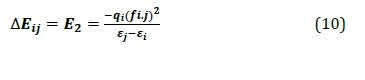Where  is the energy of the non-Lewis NBO (i.e. ),  is the energy of the orbital occupied by , and  is the occupancy of the orbital . The 'stabilization energy'  determined by second-order perturbation treatments is usually abbreviated as .

Table 7. The second order perturbation energy ( ) for a number of delocalized electrons of bonding, nonbonding and anti-bonding pairs

 NBO Donor(i) Acceptor(j) E2/ Kcal mol-1 CR(2) S(85) C(81) 1.59 S(85) C(84 1.60 S(85) C(84)-H(89) 0.94 S(85) C(81)-H(81) 0.94 BD(1) N(10)-N(20) C(81)-S(85) 0.08 N(10)-N(80) C(81)-S(85) 0.16 N(20)-N(30) C(84)-S(85) 0.31 N(30)-N(40) C(84)-S(85) 0.23 N(70)-N(80) C(81)-S(85) 0.05 LP N(10) C(81)-S(85) 0.06 N(30) C(81)-H(86) 0.1 N(30) C(84)-S(85) 0.16 N(80) C(84)-H(89) 0.09

Conclusion

In this work, the adsorptions of thiophene on zigzag (8,0) BNNT is investigated through density functional theory (DFT) calculations. These calculations indicate that the thiophene cannot be significantly adsorbed on the pristine models of boron nitride and has a poor physical adsorption on the BNNTs. The obtained results showed that the BNNTs can't detect thiophene molecules and the adsorption energy has positive value. This finding suggests that the use of structures doped with some metal atoms such as Ga-doped BNNT, Al-doped BNNT and doped nanotubes with other suitabale metals can improve adsorption. The results showed that the preferred site of molecule adsorption is at the end of the nanotube for the T fragment and the increase in polarization is due to the proper dispesion of electrons. The decrease in global hardness, energy gap, electronic and chemical potential due to thiophene adsorption leads to an increment in the stability of the (8,0) zigzag BNNT-T complex. Reduction of global hardness, energy gap, electronic and chemical potential resulting from thiophene adsorption leads to increased stability of BNNT-T zigzag complex (8,0).

Acknowledgments

Hereby, the author acknowledges Islamic Azad University, Mahshahr Branch, for supporting her to prorate software and computational instrument in informatics lab.

Conflict of interest

The author declares no conflict of interest.

Symbols

energy gap                           eV

A                             electron affinity                       eV

I                              Ionization Potential                 eV

Greek Letters

Electric conductivity of the complex

Electronic chemical potential

Global hardness

Electrophilicity index

(s)                                                          Global softness

Citation N. Farhami. A Computational Study of Thiophene Adsorption on Boron Nitride Nanotube. J. Appl. Organomet. Chem., 2022, 2(3), 163-172

###### ##### References
 A.S. Abo Dena, Z.A. Muhammud, W.M.I. Hassan, Chem. Pap., 2019, 73, 2803-2812. [Crossref], [Google Scholar], [Publisher]
 P.K. Chattaraj, U. Sarkar, D. Roy, Electrophilicity Index, J. Chem. Rev., 2006, 106, 2065-2091.  [Crossref], [Google Scholar], [Publisher]
 M. Rezaei‒Sameti, M. Jafari, M', Chem. Method., 2020, 4, 494-513. [Crossref], [Google Scholar], [Publisher]
 M. Jalali Sarvestani, R. Ahmadi, Chem. Method., 2020, 4, 40-54. [Crossref], [Google Scholar], [Publisher]
 N. Farhami, M. Monajjemi, K. Zare, Orien. J. Chem., 2017, 33, 3024-3030, [Crossref], [Google Scholar], [PDF]
 P. Geerlings, F. De Proft, W. Langenaeker, J. Chem. Rev, 2003, 103, 1793-1874. [Crossref], [Google Scholar], [Publisher]
 D. Golberg,Y. Bando, Y. Hung, T. Takeshi, ACS. Nano., 2010, 4, 2979-2993. [Crossref], [Google Scholar], [Publisher]
 R. Ma, Y. Bando, H. Zhu, T. Sato, C. Xu, D. Wu, J. Am. Chem. Soc., 2002, 124, 7672-7673.. [Crossref], [Google Scholar], [Publisher]
 N. Matsunaga, J. Comput. Theor. Nanosci., 2006, 3, 957-963. [Crossref], [Google Scholar], [Publisher]
 H. McKee, L. Herndon, J. Withrow, J. Anal. Chem., 1948, 20, 301-303. [Crossref], [Google Scholar], [Publisher]
 R. Moore, B. Greensfelder, J. Am. Chem. Soc., 1947, 69, 2008-2009. [Crossref], [Google Scholar], [Publisher]
 R. Parr, L. Szentplay, S.Liu, J. Am. Chem. Soc., 1999, 121, 1922-1924. [Crossref], [Google Scholar], [Publisher]
 A. Rodriguez Juarez, E. Chigo Anota, H. Hernandez Cocoletzi, A. Flores Riveros, Appl. Sur. Sci., 2013, 268, 259-264. [Crossref],
 A. Rubio, J. Corkill, M. Cohen, Phys. Rev B., 1994, 49, 5081-5084. [Crossref], [Google Scholar], [Publisher]
 N. Saikia, S. Pati, R. Deka, Appl. NanoSci., 2012, 2, 389-400.  [Crossref], [Google Scholar], [Publisher]
 N. Buszta, J. Wojciech Depa, A. Bajek, G. Groszek, J. Chem. Pap., 2019, 73, 2885-2888. [Crossref], [Google Scholar], [Publisher]
 N. Priyadarshini, P. Ilaiyaraja, J. Chem. Pap., 2019, 73, 2879-2884. [Crossref], [Google Scholar], [Publisher]
 R. Rashidi, J. Alenezi, J. Czechowski, J. Niver, S. Mohammad, J. Chem. Pap., 2019, 73, 2845-2855. [Crossref], [Google Scholar], [Publisher]
. P.Wang, S. Orimo, T. Matsusima, H. Fujii, J. Appl. Phys. Lett., 2002, 80, 318.   [Crossref], [Google Scholar], [Publisher]
 M. Zawari, M. Haghighizadeh, M. Derakhshandeh, Z. Barmaki, N. Farhami, M. Monajjemi, J. Comput. Theo. Nanosci., 2015, 12, 5472-5478. [Crossref], [Google Scholar], [Publisher]
 E. Tazikeh-Lemeski, A. Soltani, M.T. Baei, M. Bezi Javan, S. Moazen Rad, J. Inter. Ads. Soc., 2018, 24, 585-593. [Crossref], [Google Scholar], [Publisher]
 A.A. Peyghane, M.T. Baei, M. Moghimi, S. Hashemian, J. Clust. Sci., 2013, 24, 31-47. [Crossref], [Google Scholar], [Publisher]
 E. Chigo Anota, G.H. Cocoletzi, A. M. Garay Tapia, J. Open chem., 2015, 13, 734-742. [Crossref], [Google Scholar], [Publisher]